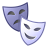# Water Cooler

This space is for everyone to ask questions related to the Community Platform. It's a space for us to get to know each other and have some fun! Come in and gather around the Water Cooler!

Announcements
QlikWorld 2023, a live, in-person thrill ride. Save \$300 before February 6: REGISTER NOW!
cancel
Showing results for
Did you mean:Contributor III

## Sum of Positive value from difference of two columns

Hi Can someone helpp me to get the output in KPI which contains the sum of only positive numbers. of 6 th Column

 Model Number Forecast Billing Quantity Forecast_2 Left Quantity (Sum(Forecast) - sum(Billing Quantity)) - (sum(Forecast_2)+sum(Left_Quantity)) Model-A 10 8 48 0 -46 Model-B 60 116 205 0 -261 Model-C 775 456 1832 0 -1513 Model-D 25 2 2 8 13 Model-E 52 59 2303 0 -2303 Model-F 80 10 20 3 47

Can we get the sum of only  positive value of  following expression in KPI:  i.e. 13+47 = 60

(Sum(Forecast) - sum(Billing Quantity)) - (sum(Forecast_2)+sum(Left_Quantity))

Thnaks

Rishabh

Labels (3)

• ### QlikView

1 Solution

Accepted SolutionsMVP

``````Sum(Aggr(
RangeMax(0, (Sum(Forecast) - Sum(Billing Quantity)) - (Sum(Forecast_2)+Sum(Left_Quantity)))
, [Model Number]))``````

4 RepliesMVP

Perhaps like this:

RangeMax(0,(Sum(Forecast) - sum(Billing Quantity)) - (sum(Forecast_2)+sum(Left_Quantity)))

talk is cheap, supply exceeds demandContributor III
Author

no its not workingContributor III
Author

hello @Gysbert_Wassenaar  your Expression works but only in Table form when I kept it in KPI then value gives 0MVP

``````Sum(Aggr(